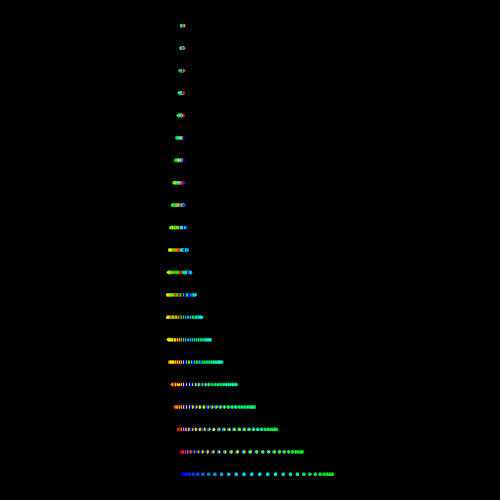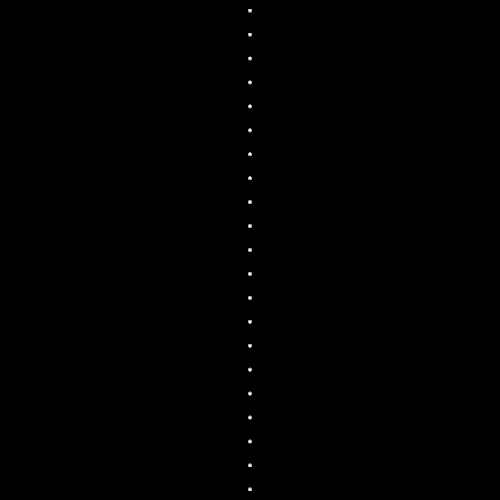# A Simple Particle Advection Example

November 20, 2014In my last blog, I introduced temporal streaming in VTK. For this, I used a simple unsteady flow-field example and at the end of the blog, I challenged the readers to developing a simple particle advection code built on temporal streaming concepts. Here is my implementation of the particle integrator.

```from source import *
from vtk.util import keys

TIME_VALUES = keys.MakeKey(keys.DoubleVectorKey, "Time Values", "particle.py")

def __init__(self):
VTKPythonAlgorithmBase.__init__(self,
nInputPorts=1, inputType='vtkDataSet',
nOutputPorts=1, outputType='vtkUnstructuredGrid')
self.Cache = None
# Seed for the particles
self.Source = vtk.vtkLineSource()
self.Source.SetPoint1(3, 0, 0)
self.Source.SetPoint2(3, 6, 0)
self.Source.SetResolution(20)
self.Source.Update()
self.NumPts = self.Source.GetOutput().GetNumberOfPoints()
# We use the probe filter to sample the input
# field at particle locations.
self.Probe = vtk.vtkProbeFilter()

# Create a polydata to represent the particle locations
# at which we will sample the velocity fields.
self.ProbePoints = vtk.vtkPolyData()
pts = vtk.vtkPoints()
self.ProbePoints.SetPoints(pts)

self.Probe.SetInputData(self.ProbePoints)

self.UpdateTimeIndex = 0

def RequestInformation(self, request, inInfo, outInfo):
# Reset values.
info = inInfo.GetInformationObject(0)
self.TimeValues = info.Get(
vtk.vtkStreamingDemandDrivenPipeline.TIME_STEPS())

# We accumulate all particles to one dataset so we don't really
# produce temporal data that can be separately requested.
outInfo.GetInformationObject(0).Remove(
vtk.vtkStreamingDemandDrivenPipeline.TIME_STEPS())
outInfo.GetInformationObject(0).Remove(
vtk.vtkStreamingDemandDrivenPipeline.TIME_RANGE())

# This is to give the next filter an idea about time
# values. Taking a shortcut here. This should really
# be a proper meta-data that is copied downstream.
outInfo.GetInformationObject(0).Set(
TIME_VALUES, self.TimeValues, len(self.TimeValues))

return 1

def RequestUpdateExtent(self, request, inInfo, outInfo):
info = inInfo.GetInformationObject(0)
# Ask for the next timestep.
info.Set(vtk.vtkStreamingDemandDrivenPipeline.UPDATE_TIME_STEP(),
self.TimeValues[self.UpdateTimeIndex])
return 1

def ProbeVelocity(self, data):
# Update the particle locations we sample at
pts = dsa.numpyTovtkDataArray(self.Points)
self.ProbePoints.GetPoints().SetData(pts)

self.Probe.SetSourceData(data)

# Sample
self.Probe.Update()
p = dsa.WrapDataObject(self.Probe.GetOutput())
# All we care about is the vector values/
return p.PointData['vectors']

def DoParticle(self, t1, t2):
# Evaluate both timesteps at the current point
v1 = self.ProbeVelocity(t1.VTKObject)
v2 = self.ProbeVelocity(t2.VTKObject)

# Use the average as the velocity
v = (v1+v2)/2
dt = t[self.UpdateTimeIndex] - t[self.UpdateTimeIndex - 1]
pts = self.Points + v*dt
self.Points = pts
# Store new particle values in the output array
idx = self.UpdateTimeIndex*self.NumPts
self.OutputPoints[idx:idx+self.NumPts, :] = pts

self.TimeValuesArray[idx:idx+self.NumPts] = t[self.UpdateTimeIndex]

def RequestData(self, request, inInfo, outInfo):
info = inInfo.GetInformationObject(0)
inp = dsa.WrapDataObject(vtk.vtkDataSet.GetData(info))

if self.Cache is not None:
self.DoParticle(self.Cache, inp)
else:
# First time step. Initialize.

# This is where we will store the coordinates of all points
# at all times
self.OutputPoints = np.zeros(
(len(self.TimeValues)*self.NumPts, 3))

# First time step uses the seed locations as the particle points
pts = vtk.vtkPoints()
pts.DeepCopy(self.Source.GetOutput().GetPoints())
self.Points = dsa.vtkDataArrayToVTKArray(pts.GetData())
self.OutputPoints[0:self.NumPts, :] = self.Points

# This will be a point array showing the time value of each
# output point. This is necessary to differentiate output
# points since we store all timesteps in the output.
self.TimeValuesArray = np.empty(len(self.TimeValues)*self.NumPts)
self.TimeValuesArray[0:self.NumPts] = self.TimeValues

if self.UpdateTimeIndex < len(self.TimeValues) - 1:
# If we are not done, ask the pipeline to re-execute us.
self.UpdateTimeIndex += 1
request.Set(
vtk.vtkStreamingDemandDrivenPipeline.CONTINUE_EXECUTING(), 1)
c = inp.NewInstance()
c.ShallowCopy(inp.VTKObject)
c = dsa.WrapDataObject(c)
self.Cache = c
else:
# Stop execution
request.Remove(
vtk.vtkStreamingDemandDrivenPipeline.CONTINUE_EXECUTING())
# Reset for next potential execution.
self.UpdateTimeIndex = 0
# Create output
outputPts = dsa.numpyTovtkDataArray(self.OutputPoints)
pts = vtk.vtkPoints()
pts.SetData(outputPts)
output = dsa.WrapDataObject(
vtk.vtkUnstructuredGrid.GetData(outInfo))
output.SetPoints(pts)
tvs = dsa.numpyTovtkDataArray(self.TimeValuesArray)
tvs.SetName("Time Values")
output.GetPointData().SetScalars(tvs)
# Clean up
self.Cache = None
self.OutputPoints = None
self.Points = None
return 1```

Most of the code in this filter actually takes care of initializing and maintaining data structures. The interesting parts are quite short. The streaming is implemented with something as simple as this:

```    def RequestUpdateExtent(self, request, inInfo, outInfo):
info = inInfo.GetInformationObject(0)
# Ask for the next timestep.
info.Set(vtk.vtkStreamingDemandDrivenPipeline.UPDATE_TIME_STEP(),
self.TimeValues[self.UpdateTimeIndex])
return 1

def RequestData(self, request, inInfo, outInfo):
if self.Cache is not None:
self.DoParticle(self.Cache, inp)

if self.UpdateTimeIndex < len(self.TimeValues) - 1:
# If we are not done, ask the pipeline to re-execute us.
self.UpdateTimeIndex += 1
request.Set(
vtk.vtkStreamingDemandDrivenPipeline.CONTINUE_EXECUTING(), 1)
c = inp.NewInstance()
c.ShallowCopy(inp.VTKObject)
c = dsa.WrapDataObject(c)
self.Cache = c
else:
# Stop execution
request.Remove(
vtk.vtkStreamingDemandDrivenPipeline.CONTINUE_EXECUTING())
return 1```

Note that we don’t do any computing in the first time step (because we need pairs of two). For all other time steps, we use the previously cached dataset together with the current dataset to do the particle integration. Also, we tell the pipeline to keep executing until the last time step is reached. Simple.

The integration code is even simpler:

```    def DoParticle(self, t1, t2):
# Evaluate both timesteps at the current point
v1 = self.ProbeVelocity(t1.VTKObject)
v2 = self.ProbeVelocity(t2.VTKObject)

# Use the average as the velocity
v = (v1+v2)/2
dt = t[self.UpdateTimeIndex] - t[self.UpdateTimeIndex - 1]
pts = self.Points + v*dt
self.Points = pts```

The ProbeVelocity function takes care of evaluating the velocity field at the given point coordinates. In this case, we evaluate both the previous and current time step at the previous particle locations. Then we average these velocities and advect the particles with this average value to obtain first order integration. In the actual VTK filter, we spent quite a bit of effort optimizing this part. Evaluating a field at a particular coordinates requires finding the cell containing it, which is non-trivial work for any grid types other than rectilinear grids.

The rest of code simply does bookkeeping. We can evaluate the filter with the following code.

```s = VelocitySource()

f.SetInputConnection(s.GetOutputPort())

renWin = vtk.vtkRenderWindow()
ren = vtk.vtkRenderer()
s = vtk.vtkSphereSource()
glyph = vtk.vtkGlyph3D()
glyph.SetInputConnection(f.GetOutputPort())
glyph.SetSourceConnection(s.GetOutputPort())
glyph.SetScaleModeToDataScalingOff()
m = vtk.vtkPolyDataMapper()
m.SetInputConnection(glyph.GetOutputPort())
m.SetScalarRange(0, 2*np.pi)
a = vtk.vtkActor()
a.SetMapper(m)
renWin.SetSize(500, 500)
renWin.Render()```

We then get the following image.I also wrote a very simple filter to animate this results. Here it is.

```from particle import *

class AnimateParticles(VTKPythonAlgorithmBase):
def __init__(self):
VTKPythonAlgorithmBase.__init__(self,
nInputPorts=1, inputType='vtkUnstructuredGrid',
nOutputPorts=1, outputType='vtkUnstructuredGrid')

self.Threshold = vtk.vtkThreshold()

def RequestInformation(self, request, inInfo, outInfo):
# We need to report that we are a time source to downstream.
# We will use the TIME_VALUES from upstream for this.
info = inInfo.GetInformationObject(0)
self.TimeValues = info.Get(TIME_VALUES)
outInfo.GetInformationObject(0).Set(
vtk.vtkStreamingDemandDrivenPipeline.TIME_STEPS(),
self.TimeValues,
len(self.TimeValues))
tr = [self.TimeValues, self.TimeValues[-1]]
outInfo.GetInformationObject(0).Set(
vtk.vtkStreamingDemandDrivenPipeline.TIME_RANGE(), tr, 2)

return 1

def RequestData(self, request, inInfo, outInfo):
info = inInfo.GetInformationObject(0)
inp = dsa.WrapDataObject(
vtk.vtkUnstructuredGrid.GetData(info))

oinfo = outInfo.GetInformationObject(0)
if oinfo.Has(vtk.vtkStreamingDemandDrivenPipeline.UPDATE_TIME_STEP()):
ut = oinfo.Get(
vtk.vtkStreamingDemandDrivenPipeline.UPDATE_TIME_STEP())
else:
ut = self.TimeValues
# Simply extract the particles where the Time Values array
# matches the requested time value.
tvs = inp.PointData['Time Values']
newpts = dsa.numpyTovtkDataArray(inp.Points[np.where(tvs == ut)])
pts = vtk.vtkPoints()
pts.SetData(newpts)

output = vtk.vtkUnstructuredGrid.GetData(outInfo)
output.SetPoints(pts)

return 1```

Very simple filter. It takes the entire collection of particles (which is essentially a collection of particle paths) and takes out the particles that belong to the requested time step. By the way, when you look at this filter, you probably understand better why I created the TIME_VALUES meta-data and the “Time Values” variable in the particle filter.\

We can now change our pipeline a bit to generate an animation. Here is the code:

```s = VelocitySource()

pa.SetInputConnection(s.GetOutputPort())

anim = AnimateParticles()
anim.SetInputConnection(pa.GetOutputPort())
anim.Update()

renWin = vtk.vtkRenderWindow()
ren = vtk.vtkRenderer()
s = vtk.vtkSphereSource()
glyph = vtk.vtkGlyph3D()
glyph.SetInputConnection(anim.GetOutputPort())
glyph.SetSourceConnection(s.GetOutputPort())
glyph.SetScaleModeToDataScalingOff()
m = vtk.vtkPolyDataMapper()
m.SetInputConnection(glyph.GetOutputPort())
a = vtk.vtkActor()
a.SetMapper(m)
renWin.SetSize(500, 500)
for time in t:
glyph.GetOutputInformation(0).Set(
vtk.vtkStreamingDemandDrivenPipeline.UPDATE_TIME_STEP(),
time)
glyph.Update()
renWin.Render()```

The output looks like this (click to animate):That’s it folks. We’ll dig into other types of streaming in the future.

Tags: